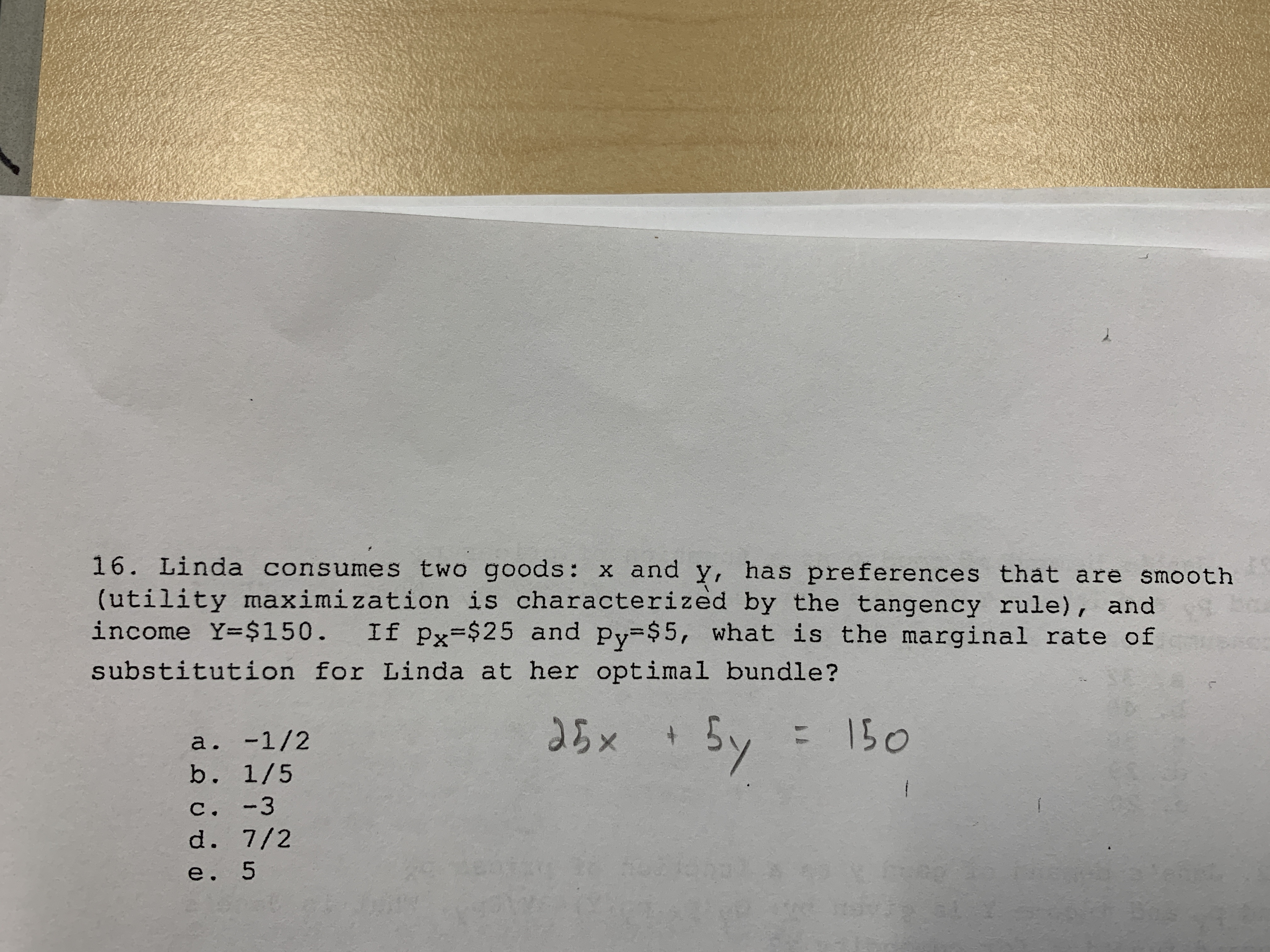# 16. Linda consumes two goods: x and y, has preferences that are smooth(utility maximi zation is characterized by the tangency rule), andincome Y=\$150.If px \$25 and py \$5, what is the marginal rate ofsubstitution for Linda at her optimal bundle?5 x5y150+a. -1/2b. 1/5C. -3d. 7/2e. 5

Question
94 viewshelp_outlineImage Transcriptionclose16. Linda consumes two goods: x and y, has preferences that are smooth (utility maximi zation is characterized by the tangency rule), and income Y=\$150. If px \$25 and py \$5, what is the marginal rate of substitution for Linda at her optimal bundle? 5 x 5y 150 + a. -1/2 b. 1/5 C. -3 d. 7/2 e. 5 fullscreen
check_circle

Step 1

Marginal rate of Substitution: In economics, the marginal rate of substitution states the point at which consumer is willing to give up one commodity for the consumption of another commodity.

Step 2

Option E, 5 is the correct answer:

Marginal rate of substitution = Px...

### Want to see the full answer?

See Solution

#### Want to see this answer and more?

Solutions are written by subject experts who are available 24/7. Questions are typically answered within 1 hour.*

See Solution
*Response times may vary by subject and question.
Tagged in